Licensed to the Apache Software Foundation (ASF) under one or more contributor license agreements; and to You under the Apache License, Version 2.0.

# Train a multi-layer perceptron (MLP) model¶

In this notebook, we are going to use PySINGA to train a MLP model for classifying 2-d points into two categories (i.e., positive and negative). We use this example to illustrate the usage of PySINGA's modules. Please refer to the documentation page for the functions of each module.

In :
from __future__ import print_function
from builtins import range

import numpy as np
import matplotlib.pyplot as plt
%matplotlib inline


To import PySINGA modules

In :
from singa import tensor
from singa import optimizer
from singa import loss
from singa import layer
#from singa.proto import model_pb2


Task is to train a MLP model to classify 2-d points into the positive and negative categories.

## Training data generation¶

The following steps would be conducted to generate the training data.

1. draw a boundary line in the 2-d space
2. generate data points in the 2-dspace
3. label the data points above the boundary line as positive points, and label other points as negative points.

We draw the boundary line as $y=5x+1$

In :
# generate the boundary
f = lambda x: (5 * x + 1)
bd_x = np.linspace(-1., 1, 200)
bd_y = f(bd_x)


We generate the datapoints by adding a random noise to the data points on the boundary line

In :
# generate the training data
x = np.random.uniform(-1, 1, 400)
y = f(x) + 2 * np.random.randn(len(x))


We label the data points above the boundary line as positive points with label 1 and other data points with label 0 (negative).

In :
# convert training data to 2d space
label = np.asarray([5 * a + 1 > b for (a, b) in zip(x, y)])
data = np.array([[a,b] for (a, b) in zip(x, y)], dtype=np.float32)

plt.plot(bd_x, bd_y, 'k', label = 'boundary')
plt.plot(x[label], y[label], 'ro', ms=7)
plt.plot(x[~label], y[~label], 'bo', ms=7)
plt.legend(loc='best')
plt.show()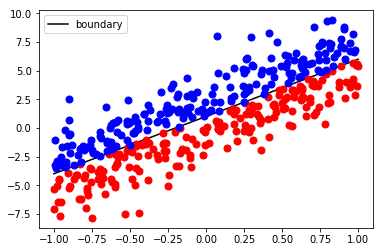## Create the MLP model¶

1. We will create a MLP by with one dense layer (i.e. fully connected layer).
2. We use the Softmax function to get compute the probability of each category for every data point.
3. We use the cross-entropy as the loss function.
4. We initialize the weight matrix following guassian distribution (mean=0, std=0.1), and set the bias to 0.
5. We creat a SGD updater to update the model parameters.

2 and 3 are combined by the SoftmaxCrossEntropy.

In :
# create layers
layer.engine = 'singacpp'
dense = layer.Dense('dense', 2, input_sample_shape=(2,))
p = dense.param_values()
print(p.shape, p.shape)

# init parameters
p.gaussian(0, 0.1) # weight matrix
p.set_value(0) # bias

# setup optimizer and loss func
opt = optimizer.SGD(lr=0.05)
lossfunc = loss.SoftmaxCrossEntropy()

(2, 2) (2,)

• Each layer is created with a layer name and other meta data, e.g., the dimension size for the dense layer. The last argument is the shape of a single input sample of this layer.
• param_values() returns a list of tensors as the parameter objects of this layer
• SGD optimzier is typically created with the weight decay, and momentum specified. The learning rate could be specified at creation or passed in when the optimizer is applied.

## Train the model¶

We run 1000 iterations to train the MLP model.

1. For each iteration, we compute the gradient of the models parameters and use them to update the model parameters.
2. Periodically, we plot the prediction from the model.
In :
tr_data = tensor.from_numpy(data)
tr_label = tensor.from_numpy(label.astype(int))
# plot the classification results using the current model parameters
def plot_status(w, b, title='origin'):
global bd_x, bd_y, data
lbl = pr[:, 0] < pr[:, 1]

plt.figure(figsize=(6,3));
plt.plot(bd_x, bd_y, 'k', label='truth line')
plt.plot(data[lbl, 0], data[lbl, 1], 'ro', ms=7)
plt.plot(data[~lbl, 0], data[~lbl, 1], 'bo', ms=7)
plt.legend(loc='best')
plt.title(title)
plt.xlim(-1, 1);
plt.ylim(data[:, 1].min()-1, data[:, 1].max()+1)

# sgd
for i in range(1000):
act = dense.forward(True, tr_data)
lvalue = lossfunc.forward(True, act, tr_label)
dact = lossfunc.backward()

dact /= tr_data.shape
_, dp = dense.backward(True, dact)

# update the parameters
opt.apply(i, dp, p, 'w')
opt.apply(i, dp, p, 'b')

if (i%100 == 0):
print('training loss =  %f' % lvalue.l1())
plot_status(tensor.to_numpy(p), tensor.to_numpy(p),title='epoch %d' % i)

#train(dat, label)

training loss =  0.704490
training loss =  0.483168
training loss =  0.411447
training loss =  0.362376
training loss =  0.326843
training loss =  0.299900
training loss =  0.278710
training loss =  0.261552
training loss =  0.247332
training loss =  0.235318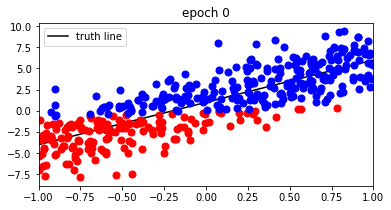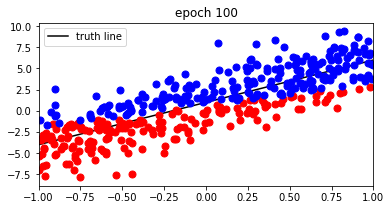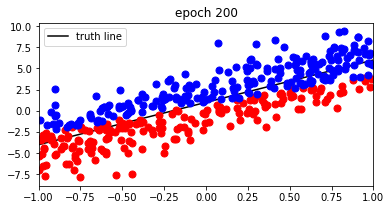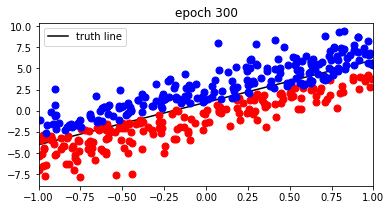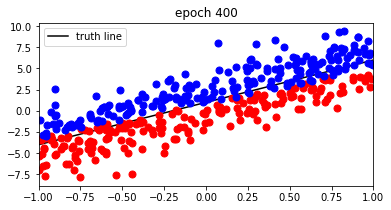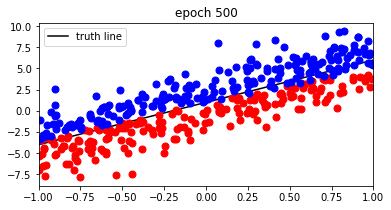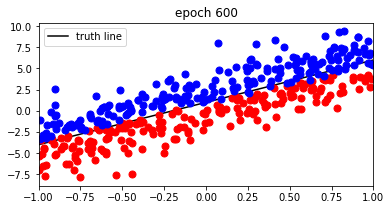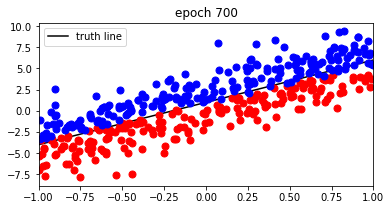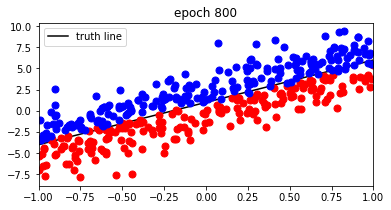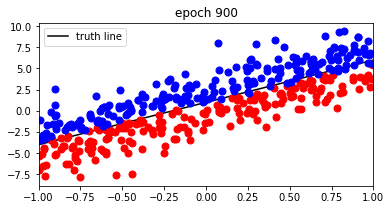The layer class has forward and backward functions for back-propagation.

• forward() accepts two arguments, the first one indicates the phase (training or evaluation); the second one includes the input tensor(s); It outputs the layer values as a single or a list of tensors.
• backward() accepts two arguments, the first one is not used currently; the second one includes the gradients of the layer values. It outputs a tuple, where the first field includes the gradient tensor(s) of the input(s), and the second field includes a list of gradients for the parameters.

The optimzier class apply function updates the parameter values using the gradients. The first argument is the iteration ID, followed by the gradient tensor and the value tensor. Each parameter tensor has a name associated with it, which is used by Optimizer to keep some internal data (e.g., history gradients) for each parameter.

The loss class computes the loss value given the predictions and the ground truth in forward() function. It computes the gradients of the predictions w.r.t the loss function and outputs the gradient tensor(s) by backward() function.

## Observation¶

We can see that prediction of the data points are getting correct labels with the training going on.